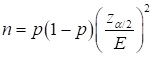# 4. A researcher wants to calculate the prevalence of Alzheimer’s among members of a specific community who are 65 years of age and older. The researcher needs to know how many individuals who are 65 years of age or older need to be recruited for the study to ensure the estimated prevalence will be within 1.0% of the true proportion. Calculate the sample size needed by the researcher if a national study has shown 11.11% of individuals 65 years of age have Alzheimer’s and the researcher wants to be 95% confident of the estimate.A) n = 3,794B) n = 2,673C) n = 6,549D) n = 152

Question
29 views

4. A researcher wants to calculate the prevalence of Alzheimer’s among members of a specific community who are 65 years of age and older. The researcher needs to know how many individuals who are 65 years of age or older need to be recruited for the study to ensure the estimated prevalence will be within 1.0% of the true proportion. Calculate the sample size needed by the researcher if a national study has shown 11.11% of individuals 65 years of age have Alzheimer’s and the researcher wants to be 95% confident of the estimate.
A) n = 3,794
B) n = 2,673
C) n = 6,549
D) n = 152

check_circle

Step 1

Sample Size:

The formula for the sample size is as follows:Here, zα/2 is the critical value at 95% confidence level, p is the population proportion and E is the margin of error.

Step 2

Computing the sample size:

The confidence level is 95%.

Therefore, α = 0.05.

In this case, α/2=0.025, 1– α/2 = 0.975.

Using the standard normal table, the critical value for a level of significance 0.05is 1.96.

The estimate of true proportion is within 1.0%.

From the given information E = 0.01, p= 0.1111 and zα/2 =1.96.

Then...

### Want to see the full answer?

See Solution

#### Want to see this answer and more?

Solutions are written by subject experts who are available 24/7. Questions are typically answered within 1 hour.*

See Solution
*Response times may vary by subject and question.
Tagged in

### Statistics